# Cost of Debt

It is the effective interest rate a business pays on its obligations to creditors and debtholders

The cost of debt is the effective interest rate a business pays on its obligations to creditors and debtholders. It is an expected rate of return for the companies that provide credits and debt and is expressed as a percentage.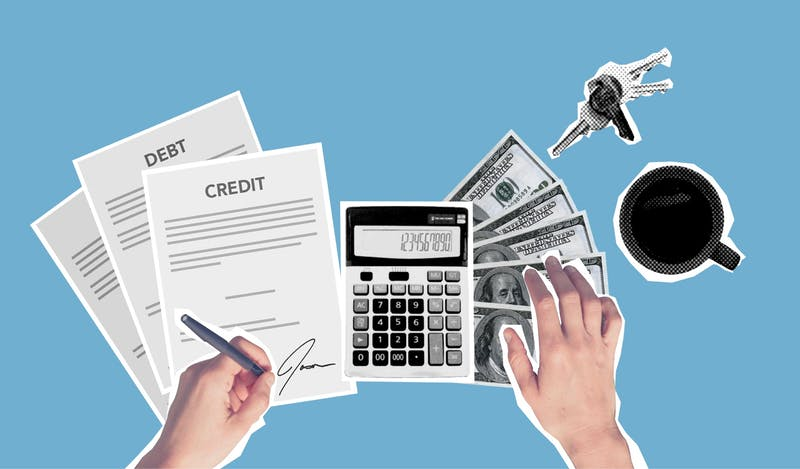It is a crucial component of discounted valuation analysis, which determines a company's present value by dividing predicted future cash flows by the expected rate of return on Equity and debt.

It is expressed as either the before-tax (the amount owed by the business before taxes) or the after-tax. Because interest costs are tax deductible, there is a significant difference between the debt cost before and after taxes.

Interest expenses are deductible from taxable income, resulting in the firm's savings that are available to the debt holder.

• A company's before-tax debt cost represents the entire interest expenditure the company spends in any given year.

• The expected rate of return (before tax) represents the total interest expenses upon the company's debts/ credits.

• A company's after-tax debt cost is represented by deducting the tax from the interest cost. Therefore, it is considered in the DCF methodology for determining the effective interest rate.Debt is a vital component of a company's capital structure in terms of using various funding sources to fund its operations and keep the business growing. Therefore, companies should understand how much they need to pay for debts to determine if they can pay all debt costs.

Debt cost includes the amount the company borrows with interest required by the creditors or bondholders. Determining the debt cost helps companies determine the rate of money paid by the company to finance its debt regularly.

Therefore, the company can determine the risk they take to finance its debts and loans compared with other companies in the market. Companies with the highest debt cost are known as risky companies.

The financial analysts or investors could determine the company's risk level by looking at the cost of its debts compared with other companies in the same field. An increased cost debt rate could decline borrowers' credit health since the lending risk increases.

On the other hand, a decrease in the rate of cost debt strengthens the borrowers' credit health since the lending risk goes down, which is associated with low-risk companies.

## Cost of debt formula

It is a rate of return demanded by debt holders to take the risk of lending money to a particular borrower.

In other words, It is the total of the effective interest rate that a business must pay on its long-term debt obligations and the minimum yield lenders want to make up for the potential capital loss when making a loan to a borrower.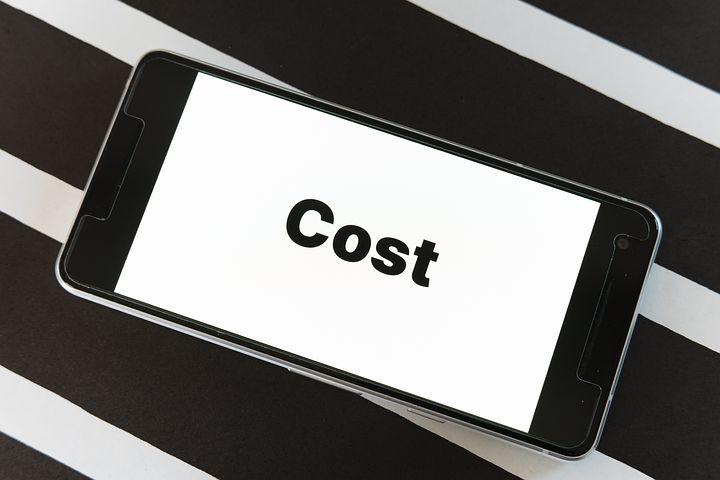There is more than a way to calculate this measure regarding pre-tax or post-tax cost of debt.

If you want to calculate the pre-tax cost, use the following formula: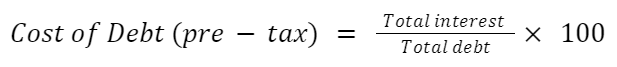To calculate the post-tax, use the following formula: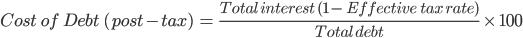Or: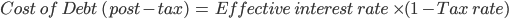As shown in the previous formula, these three metrics should be determined to determine the cost associated with acquiring a debt. The three components are as follows:

1. Total interest: The total interest cost incurred by a company in a year.

2. Total debt: Total debt at the end of the fiscal year.

3. Effective tax rate: The average percentage of a company's profits paid in taxes.

For instance, suppose a company had a \$200 long-term loan with a 5% annual interest rate and a \$300 bond with a 6% annual interest rate. Then, the pre-tax debt cost is calculated as follows: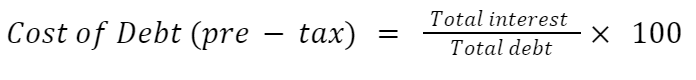• Notice that total interest is calculated by multiplying the total debt by the interest rate. The interest rate is determined by the party that offers the obligations to borrowers.

= ((\$200 * 5%) + ( \$300 * 6%) / (\$200 + \$300)) * 100

= ((\$10+ \$18) / (\$500)) *100

= 0.056 * 100

= 5.6%

Hence, the pre-tax cost of a loan is 5.6%. Unfortunately, you cannot calculate the post-tax cost of loans since the tax rate isn't given in the company's information.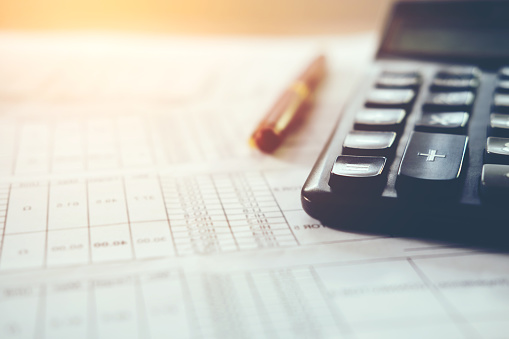## Examples

Let's see some examples to understand how to apply the formula best.

1. A company has a \$1000 bond repayable in 6 years at an interest rate of 7% with 35% an effective tax rate. What is the pre-tax and after-tax cost of debt?

The yearly interest expense incurred by the company in one year is:

Interest expense= Total debt * Interest rate

= \$1000 * 7%

= 70\$

Hence, the interest expense that companies pay in one year is 70\$.

The pre-tax debt's cost is: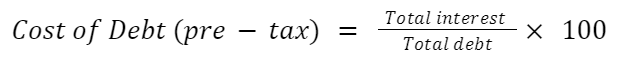= (70\$ / \$1000) * 1000

= 0.07 * 100

= 7%

Suppose that the company deducts 20\$ from the taxable income, the net tax would be 70\$ - 20\$ = 50\$. The post-tax debts cost is calculated as follows: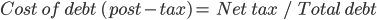= (50\$ / \$1000) * 1000

= 0.05 * 1000

= 5%

Or:= (70\$ * ( 1- 35%) / \$1000) * 100

= (70\$ * ( 0.65) / \$1000) * 100

= (45.5 / \$1000) * 100

= 0.0455 * 100

= 4.55 ~ 5%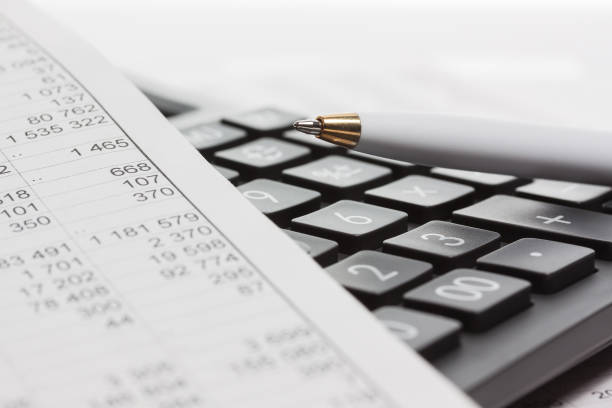2. A company has a \$1000 bond repayable in 3 years at a coupon rate of 8% with 20% of an effective tax rate. What is the pre-tax and after-tax debt's cost if the interest is paid semi-annually?

Let's first figure out the interest rate:

interest rate = (Coupon rate/ 2) * Total bond

= (8% / 2) * 1000

= 4% * 1000

= \$40

Hence, the company paid 40\$ two times in one year. In other words, the total interest expense in one year is \$80.

Let's figure out the debt cost before taking into consideration the tax impact: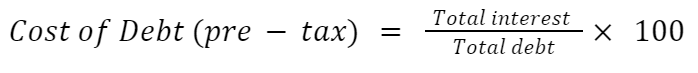= (\$80 / 1000) * 100

= 0.08 * 100

= 8%

Now, Let's figure out the debt cost after taking into consideration the tax impact:= \$80 * (1- 0.20) / \$1000) * 100 = (\$80 * 0.8 / \$1000) * 100

= (64 / 1000) * 100

= 0.064 * 100

= 6.4%

## Advantages in determining the Cost of Debt

Although many people see debt as a wrong financial tool, business owners and finance directors understand the importance of debt. In addition, business owners know how to use debt as a tool for business expansion.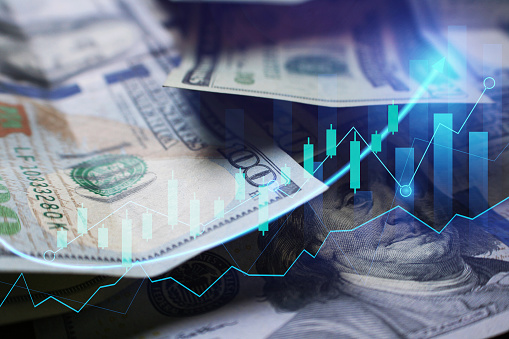Therefore, the company gets inaccurate information about its debt obligation and ability to pay back the debt amount.

There is no doubt that debt is an important way for many businesses to get funding. Companies use loans and credit cards to run their businesses and improve their operations. Taking debt is a vital step almost every industry takes to be competitive in the market.

The advantages of determining the debt cost are as follows:

1. Determine the company risk level

Figuring out how much a business is paying for its debt is an excellent way to determine how risky a company is and how well it can handle other credit and loans without downgrading its credit health.

Therefore, investors and financial analysts could compare companies in the same field if they desire to invest in a particular company.

Keep in mind that an increase in the cost debt rate leads to a decline in borrowers' credit health because the lending risk increases.

2. Determine the company's profits

Every business should keep track of what debts they still owe and how much debts cost. Then, after excluding all debt expenses, it is vital to figure out how much money it makes.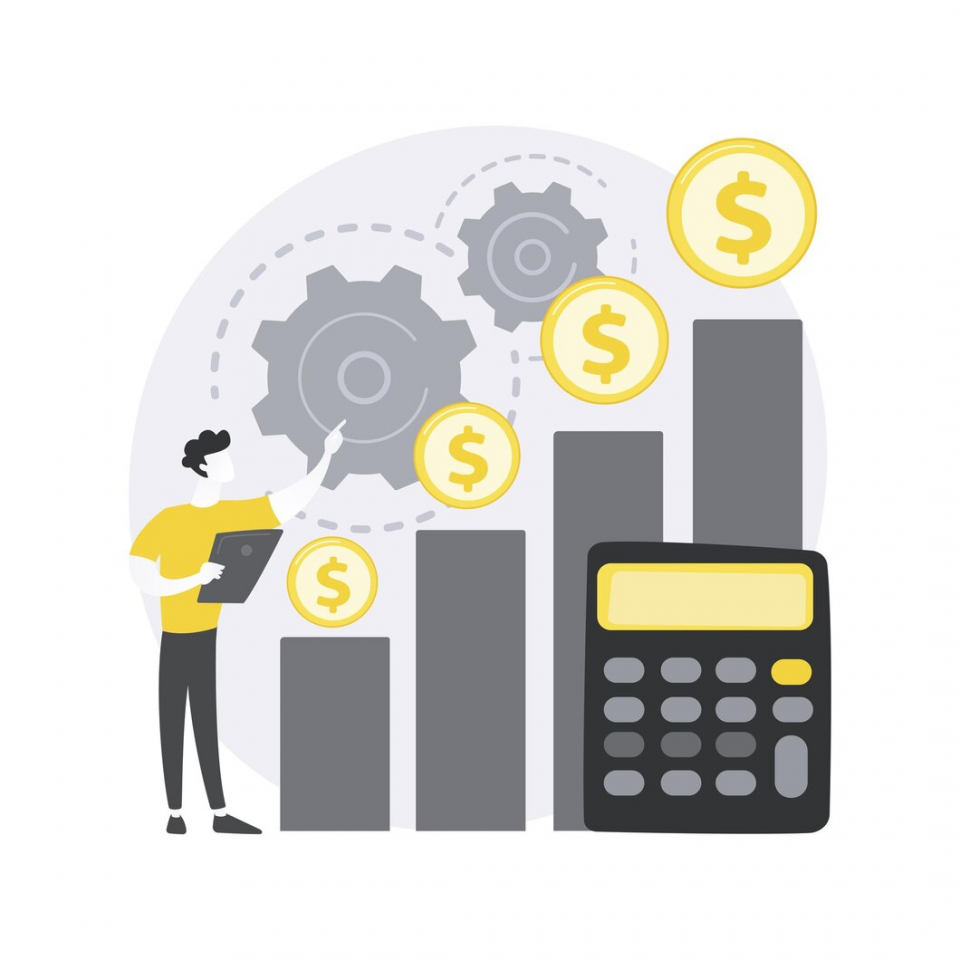It also tells you how much money a company has to make to pay its debt obligations. This information gives businesses valuable financial insights they can use to improve their financial situation and create a better plan to fulfill their financial obligations.

3. Determine the best funding source

It gives a good idea of the adjusted rate the companies pay and helps them decide whether to use debt or equity funding.

By comparing the debt cost to the expected growth in income from the capital investment, it would be possible to get a clear picture of the overall returns from the funding activity.

## The differences between the cost of debt and Equity

The cost of Equity and debt are the two most essential elements in the price of capital. Companies can acquire money through Equity or debt, with the majority preferring a combination of the two.The critical difference between these sources of funding is the cost of capital is the required rate of return on shareholders' investments. On the other hand, debt costs are provided for debt holders.

Unlike debt financing, equity financing is a financial instrument without a fixed rate of return. Investors buy stock in a firm with the hope that they will get dividends in the future as their investment will grow over time.

The Capital Assets Pricing Model (CAPM) is one of the most commonly used models for calculating the cost of Equity. This model looks into the relationship between systematic risk and expected return on assets, specifically stocks.The key differences between the debt and equity costs are as follows:

1. Rate of return

The rate of return on Equity is the rate of return expected by the shareholders of their investment.

The rate of return on debt is the rate expected by the bondholders of their investment.

2. Interest

Equity cost isn't tax deductible since it doesn't pay interest regularly.

Debt cost is tax deductible (tax saving) since it pays interest regularly.

3. Cost

• Equity: Dividends paid to equity shareholders are more expensive than debt interest.

• Debt: interest paid to bondholders on debt is lower than the dividends paid to equity shareholders.

The mix of debt and Equity is vital for a company and should always be at a reasonable level. However, there is no metric for how much debt a company should have compared to its Equity.

The most crucial point is that the company should be aware of the equity and debt costs.

There is no lending risk as long as the company can handle its operations and generate the money required to pay back to shareholders or bondholders.

## Key Takeaways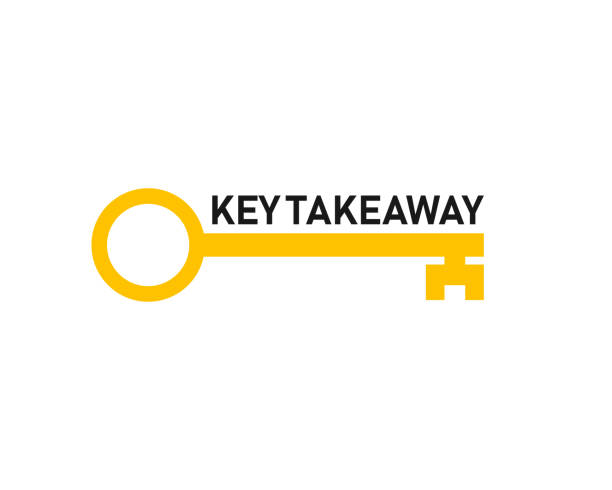• The cost of debt is the effective rate of interest a company pays on its loans to creditors and debtholders. It is an estimated rate of return given as a percentage for organizations that provide credit and debt.

• It can be expressed as either the cost of debt before taxes (the amount owed by the business before taxes) or after taxes.

• Some advantages of determining the debt cost determine the company's risk level and profits by its total cost of debt.

• This cost of debt shows limitations since it is calculated without considering how a company's capital structure changes over time. So, the company gets inaccurate insights about how much debt they owe and its repayment capacity.

• The critical difference between the cost of Equity and debt is the cost of Equity is the required rate of return on shareholders' investments. On the other hand, the debt cost is the rate of return expected by the bondholders of their investment.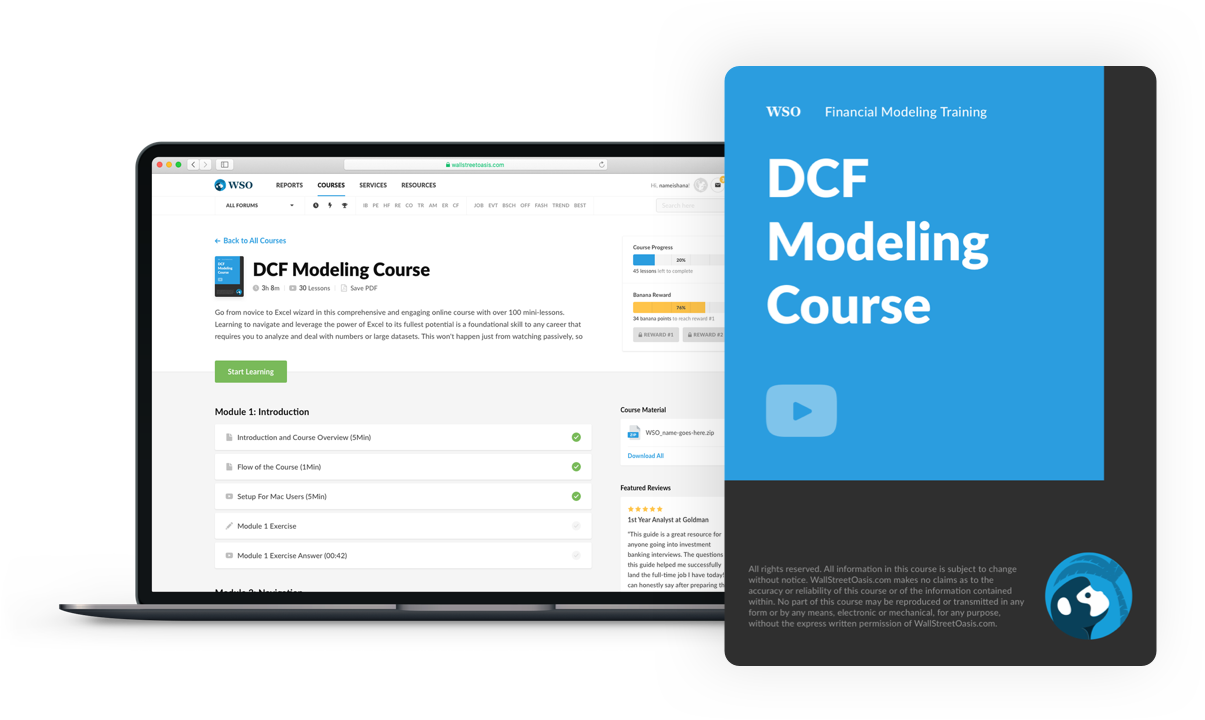### Everything You Need To Master DCF Modeling

To Help You Thrive in the Most Prestigious Jobs on Wall Street.